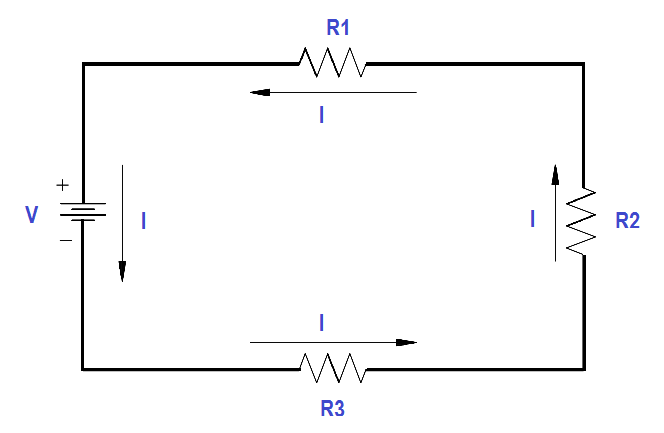# Series Circuit

A series circuit is a circuit where there is only one path for current flow.

In a series circuit (Figure 16), the current will be the same throughout the circuit. This means that the current flow through R1 is the same as the current flow through R2 and R3.Figure 16 Series Circuit